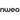# How to Teach Multiplication in 6 Easy Steps

All Posts

The leap from learning subtraction and addition to learning multiplication is one of the most daunting tasks students will face at school.

And it’s not just students who have trouble with the subject.When teaching multiplication, educators frequently start with the wrong concepts or work through lessons too quickly. This can discourage and intimidate students, ultimately damaging learning outcomes. Thankfully, there are known strategies to avoid these obstacles. Use the guide below to see how you can make teaching and learning multiplication easy in six simple steps that will engage and resonate with students. This article is broken down into two sections:
 Section A A six-step guide to teaching multiplication ✔Learn how to introduce key concepts and how to teach kids to learn and memorize the multiplication table! Section B Fun ways to learn multiplication ✔A list of fun ideas for teaching multiplication to your students!

## 1. Relate multiplication to addition

Avoid starting with memorization. Students typically struggle to memorize multiplication facts on the first try, and this can lead to a fear of the multiplication table. The simplest way to begin teaching multiplication is to anchor the concept in terms of its relation to addition -- an operation your students should already be comfortable with.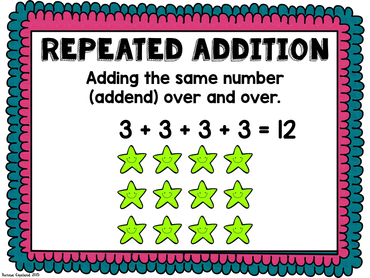Before moving on, ensure your students grasp the first pillar of multiplication: that it is simply repeated addition. Use relatable examples, like the one above, to contextualize this fact.It will help to write out examples so students can visualize the idea:

2 x 2 is the same as 2 + 2

or

3 x 4 is the same as 4 + 4 + 4

### Multiplying by zero: The zero property

Highlight to your class that adding zero to a number has no effect on its identity:

n + 0 = n

Next, explain that in multiplication, any number multiplied by zero is also zero:

n x 0 = 0

Encourage students to discover examples of the zero property in the room. For example, a classroom that has 25 chairs with zero monkeys sitting on each one means that there are no monkeys in the classroom.Optionally, one amusing example of a fun math activity to entertain your students involves thinking of funny examples of this property, like 0 x one million = 0 ... or ... 0 x ham sandwich = 0. [caption id="" align="aligncenter" width="351"]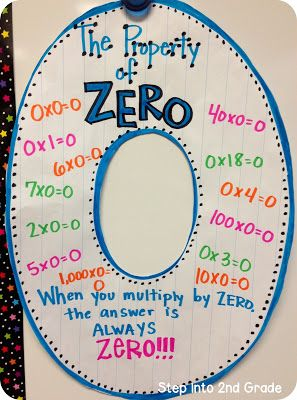Image source: Step Into 2nd Grade[/caption]

### Multiplying by one: The identity property

In short, the identity property states that the product of a given number and one is that number itself: n x 1 = n.Just like with the zero property, highlight to your class that multiplying a number by one results in the same value. To ground the property in a real-life context, encourage your class to think of instances of the identity property in the classroom.For instance,
• One group of eight desks is eight desks
• A single row on the calendar showing seven days is seven days
[caption id="attachment_2000" align="aligncenter" width="386"]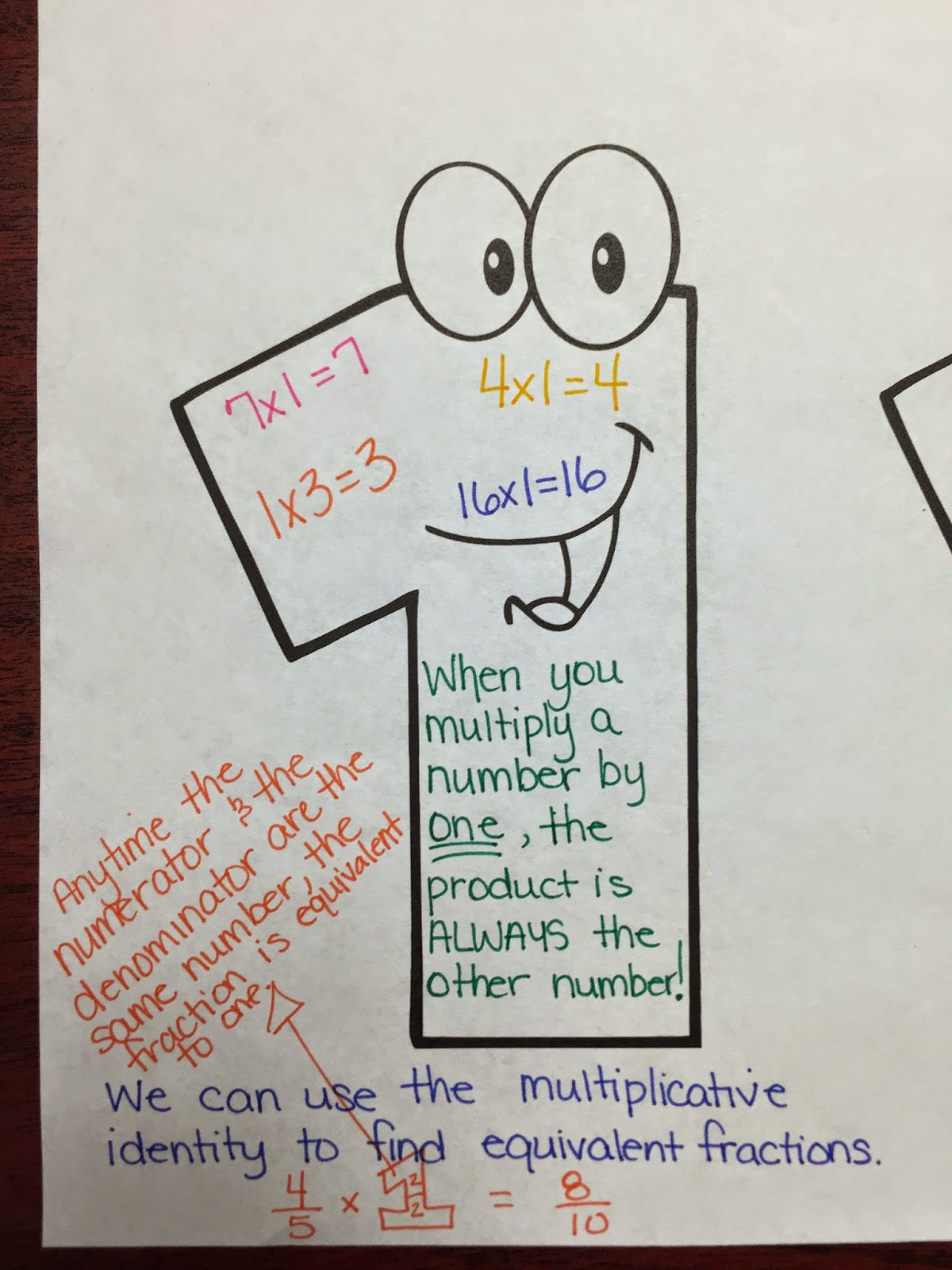Image source: The Classy Teacher[/caption]

## 3. Cover the multiplication table, starting with the “easy” numbers

To review, your students should now understand that multiplication can be thought of as repeated addition. They should also have taken time to multiply numbers by zero and one. While they don’t need to know the technical terms, students should understand how the zero property and identity property work.Now, it’s time to take on one of the biggest leaps: covering the multiplication table.One effective approach works as follows:
• Display a chart or overhead slide of the 12 x 12 multiplication table. If you wish, supplement this with student copies of the multiplication chart. If a 12 x 12 chart seems too overwhelming, perform the same process with a 10 x 10 multiplication chart.
• Teach students how to use the chart and find products by following and matching the position of numbers using the vertical and horizontal axes.• Now, talk about some of the patterns you can find in the chart. Offer students clues or hints. For example, note how every multiple of ten ends in zero, and every multiple of five ends in zero or five.
• Ask students which facts are the easiest. For instance, the ones and tens are easy. Now that you have discussed the "easy" multiplication facts -- and what it is that makes them easy -- eliminate them from the chart.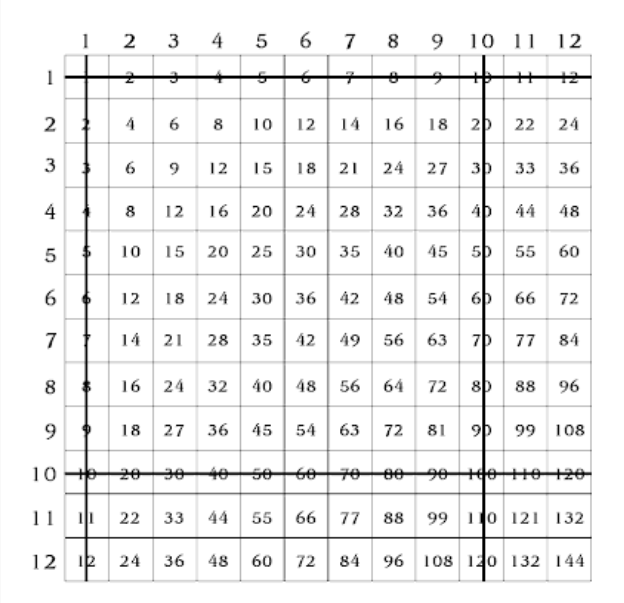Students usually agree that the twos and fives and elevens are also easy. Continue crossing numbers the “easy” numbers out, until you arrive at a stage that looks something like this: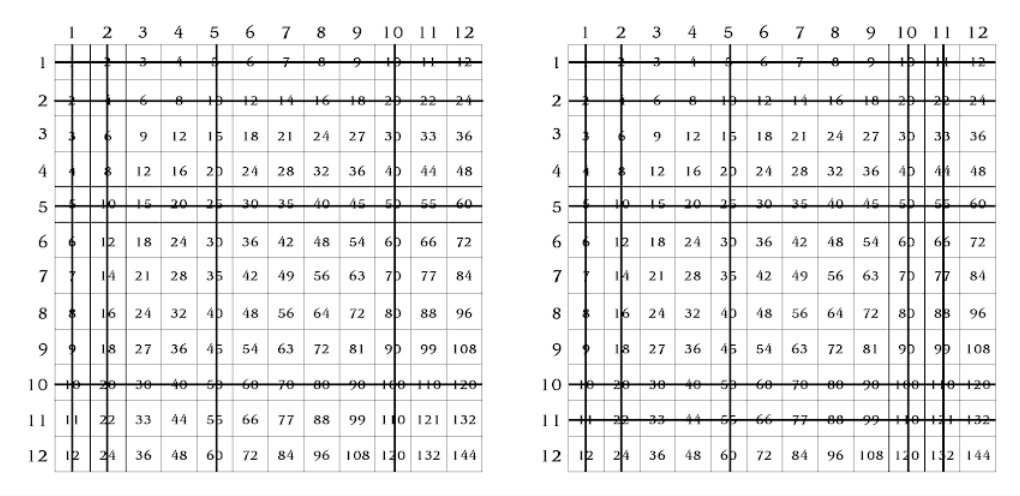• Look at the remaining numbers with your class. Use the opportunity to lead a dynamic discussion and lead into the next step.

## 4. Show how the commutative property makes things easier

Just like addition, multiplication is commutative, meaning the order of factors doesn’t change the product (the answer). In other words, two numbers can be multiplied in any order, and the product will be the same. For example, multiplying 8 x 2 will give you the same answer as multiplying 2 x 8. When you communicate this effectively, it will encourage your students.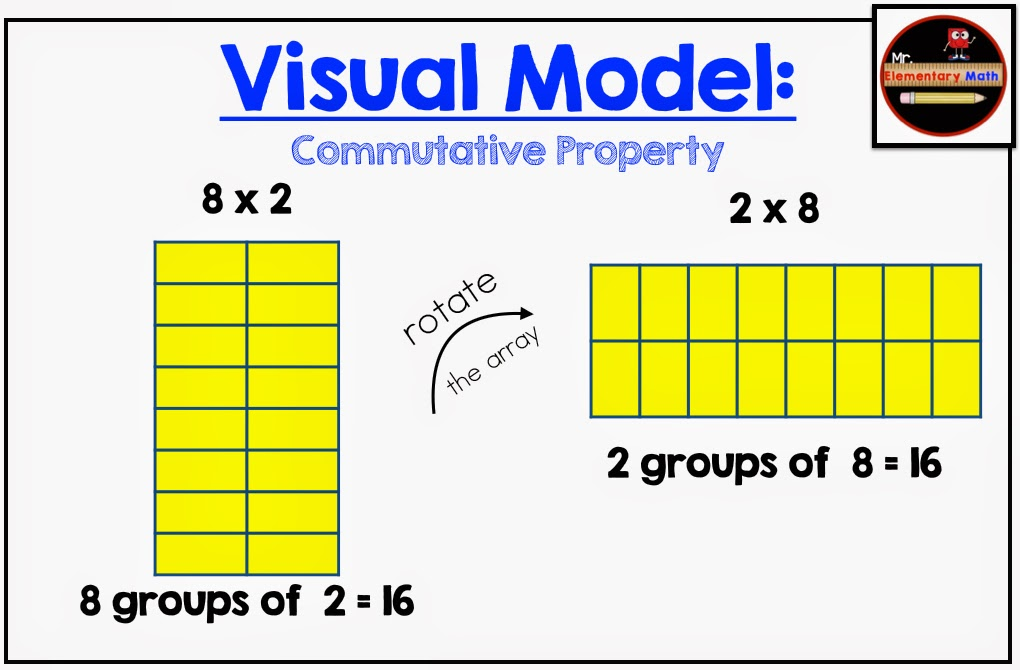Show your students that each answer repeats, so they only have to learn half of the remaining tables. For example, explain that learning 3 x 6 is the same as learning 6 x 3! If students have already learned the fact families of 0-3, then they also know four numbers each of 4-10.To further reinforce the commutative property, you can also use a visual model like the one above and point out how the yellow blocks are arranged.Ask your students, “aren't these two arrangements the same, but just different versions of each other? If we count the total number of units, are they the same?”Helping students grasp this property is a key part of teaching multiplication. To get students to do it themselves and learn through direct experience, have your class create models to illustrate this idea, and prove that a x b results in the same product as b x a.

## 5. Break memorization down into easy steps

At this point, your students are ready to begin memorizing the multiplication table. It is likely that they have already begun to do so. Be aware that it is not always obvious to students why they need to learn multiplication facts off by heart. Communicate the importance of understanding the meaning behind multiplication facts and how they can be used in everyday situations.While there are many approaches to memorizing the multiplication chart, recall the examples above -- the “easy” numbers -- as a good starting point. As you continue your lesson, consider following these rules:
• Encourage students and set time for them to practice verbally or in writing.
• Introduce new multiplication facts one by one, gradually and incrementally opening the concept to the more advanced steps of multiplying by 2, 3, 4 and so on.
• Give students time to practice the multiplication facts. Find a rhythm that works well in your classroom. If you wish, take advantage of peer learning for students who are struggling.
• Memorize the facts in a strategic order. Once your students master 0-3, proceed to 4-7, and then 8-10. Depending on your preference, you may also cover 11 and 12. Including more challenging problems as a bonus will help you to gauge high-achieving students. Some teachers will include a few harder problems for a bonus or to identify high-achieving students.
To help with memorization, keep visual materials on hand in your classroom. No matter how many times you cover multiplication, it’s always a good idea to have a physical copy of the table available in your classroom. If you don’t have one, you can order one online for less than 10 dollars. A quick search on Amazon shows an array of options.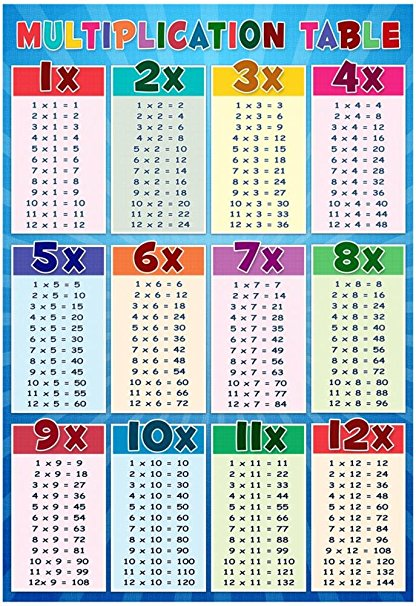If you already have a poster, consider getting other materials that will help you explain the function of multiplication visually. For example, this document is a great option for displays: it contains a 20 page set of answers (like the one seen below) to each of the times tables from 1-10, color coded according to the specific table they represent.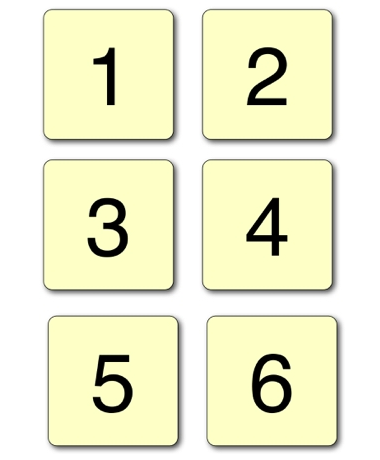## 6. Introduce the associative and distributive properties

While these concepts are more advanced, it is important your students grasp them. You will find they can work wonders to improve your students’ mental math. Note: Use your discretion when introducing the properties below. They may not be applicable to students in any year lower than the 4th Grade.

### The associative property

The rule of associative property states that no matter the way you group factors, the product will always be the same. For example,

(a x b) x c = a x (b x c)

or

(1 x 2) x 3 = 1 x (2 x 3)

Your students can understand this concept by relating it to column addition, whereby they group addends to find the sum. Students can also learn to group factors in any way they find most convenient as they look for the answer. You may explain this rule as an extension of the commutative property above.

### The distributive property

The distributive property is symbolized as a(b + c) = (ab) + (ac)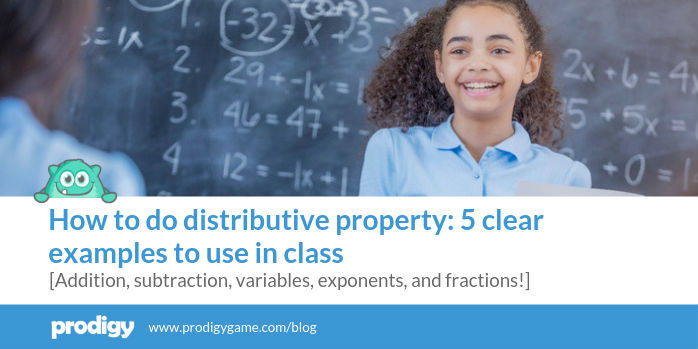That might look tricky to students, but frequently they are already using the property by themselves as a mental math trick to solve more challenging multiplication facts. Put simply, the property spreads out -- or, as its name implies, distributes -- the value of a equally to b and c. For example, for 6 x 8, they may use the distributive property to think: (6 x 5) + (6 x 3), or 30 +18To explain the property to visual or tactile learners, allow children to explore the distributive property with materials such as cups and beans or grid paper.

## Play Classroom Math Games

Classroom math games can take the fear out of multiplication. Consider these two examples specialized to multiplication:Calculator Constants If the calculators in your classroom have a constant function, encourage your students to use them to discover the multiples of any number to find the link between multiplication and addition.How it works:
• Pick a factor such as seven, and enter it.
• Hit the + button, then press the = button. The calculator should show seven again.
• Next, continue to press the = button. The constant function will continually add seven, showing the number’s incremental multiples (7, 14, 21, 28, and so on)
• Optionally, have students record a list of the multiples they find and encourage them to look for patterns in the list they come up with
Times Table Buzz This is a fun math activity to motivate your students, reinforcing their knowledge of the multiplication table.Step a) Pick a specific multiplication table to use for the activity (for example, multiples of five)Step b) Have your students stand up and arrange them in a certain order, such that each student knows when it’s their turnStep c) Choose one student to count “one.” Next, the child to his or her left counts “two.” The following child counts “three,” and so on. When the sequence reaches a multiple of five, the student shouts out “buzz!” Therefore, the sequence will proceed as: one, two, three, four, “buzz!”, six, seven...Step d) If a student forgets to say “buzz” or says it at the wrong time, they are out and sit down. The counting sequence will continue until the students reach ten times five (or at the teacher’s discretion) and will then start again.The final winners are the last three children standing. Consider rewarding the winners as part of your classroom management strategy.For more inspiration, review our full list of 20 engaging, skill-building classroom math games. For an active approach to teaching math, review our 20 fun and creative math activities -- approaches and exercises, with and without computers, that will make students look forward to your math lessons.

## Use fun math books in class

In our review of math books for students, we found a list of exceptional resources for teachers. Review numbers three, five, and six to find examples of publications that feature multiplication and help support a well-structured math curriculum.

## Support learning with math websites

The internet is home to a vast selection of powerful and useful educational math websites for teachers and students. See our list of helpful math websites for teachers, five of which you can share with students.One resource is Prodigy, a free, curriculum-aligned math game used by more than 800,000 teachers and 30 million students around the world.
Prodigy offers content from every major math topic -- including multiplication -- and covers 1st to 8th Grade. You can make learning multiplication a fantasy-inspired journey where students’ success depends on honing curriculum-aligned math skills!It’s also backed by quantitative research. In a recent study, we found that schools enrolled in Prodigy both performed better and enjoyed greater improvements on test results than inactive schools.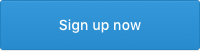## How to Teach Multiplication: Final Thoughts

If multiplication is a science, then helping your students understand the operation is an art.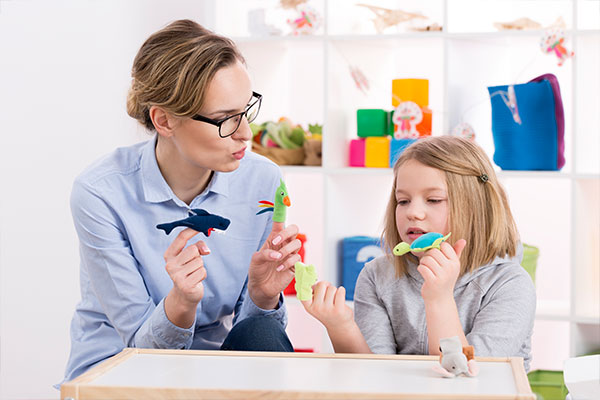With these strategies and tools in mind, you can see how multiplication doesn’t have to be a scary or daunting subject -- for yourself or for your students. When broken down into digestible constituents, you will avoid the frustrations that frequently accompany the practice of learning multiplication. Use these ideas to reinforce a well-structured math curriculum, and watch your students’ learning outcomes improve.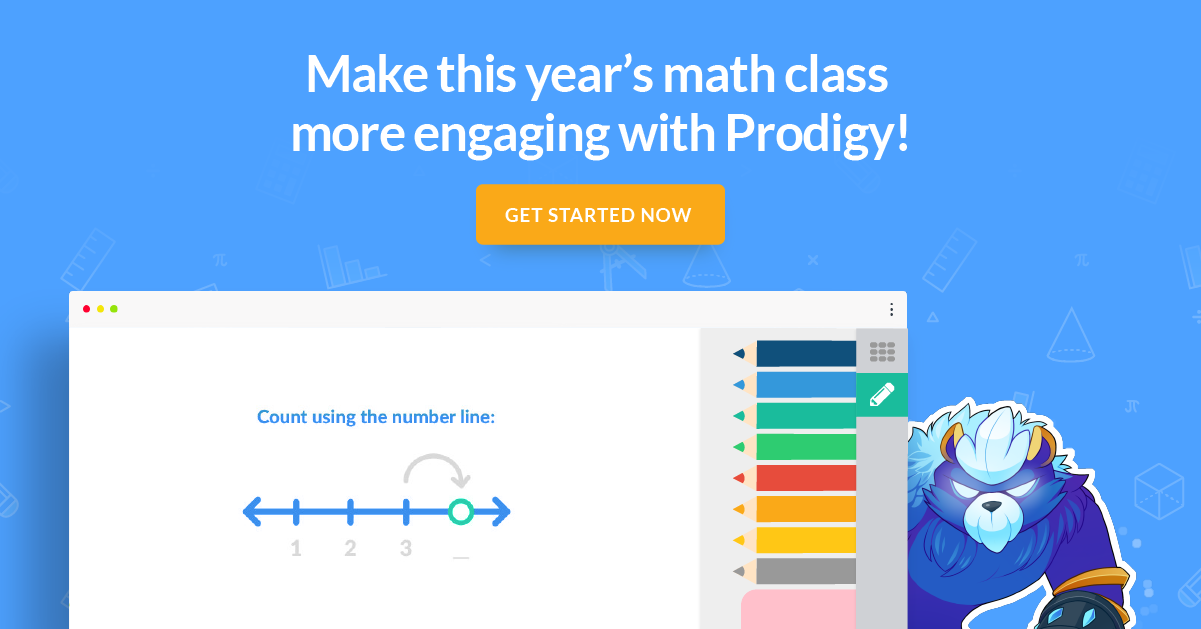•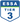•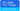•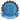•